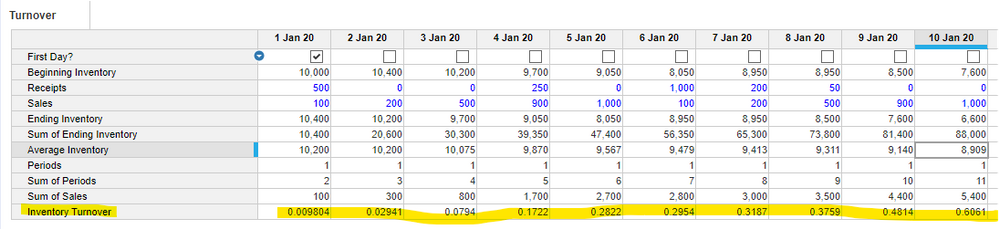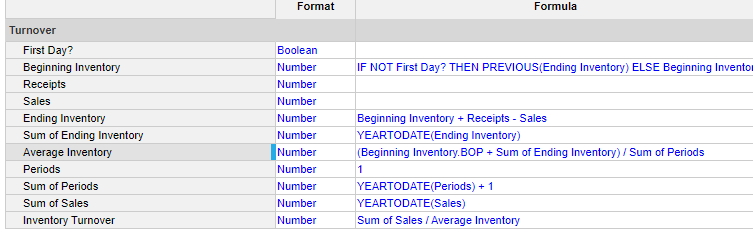# How to calculate the turnover rate?Hi, I have a question about calculating the turnover rate.

To calculate it, I use this formula.

Formula:

IF Inventory <= 0 THEN 0 ELSE IF Inventory <= MOVINGSUM(Demand, 1, 1) THEN 1 ELSE IF Inventory <= MOVINGSUM(Demand, 1, 2) THEN 2 ELSE IF Inventory <= MOVINGSUM(Demand, 1, 3) THEN 3・・・・

But this formula continues forever if I want Turnover rate = 30.

Does any body has any solution?

 2021/1/1 2021/1/2 2021/1/3 2021/1/4 2021/1/5 Demand 0 200 300 200 100 Inventory 600 400 Turnover rate 2day 1day 30day

Tagged:

•You can calculate your end period for formula in a separate line item which will reduce your length of formula. Use the day(Date formatted line item) function to figure out the day. now you will be able to recreate your formula like this IF Inventory <= MOVINGSUM(Demand, 1, DAY) THEN DAY ELSE 'OTHER CONDITION'

Thanks

Abhay

•I would like to add more to @abhay.kanik answer and perhaps stretch you a little bit to consider making this a bit more formal.

Your question seems to be more aligned with a "Days of Supply". Which takes the inventory and divides by an average daily sale. That might be more intuitive to you.

If you're determined to calculate a turnover, the best way I know of to calculate an inventory turnover is using these equations:

Turnover = Sum(Sales) / Average Inventory

Average Inventory = [Beginning Inventory + Sum(Ending Inventory)] / Number of Periods + 1

Here's an example in Anaplan. Also, try to keep an inventory balance set for accuracy. Ending = Beginning + Receipts - Sales.